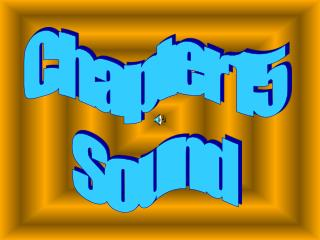DownloadDownload PresentationChapter 15 Sound

# Chapter 15 Sound

Download Presentation## Chapter 15 Sound

- - - - - - - - - - - - - - - - - - - - - - - - - - - E N D - - - - - - - - - - - - - - - - - - - - - - - - - - -
##### Presentation Transcript

1. Chapter 15 Sound

2. 15.1 Properties of Sound The production of sound Waves always begin with A vibrating object.

3. This vibration causes the Molecules around it (usually Air molecules) to move. This movement is a wave pattern. Compression is the region of a Longitudinal wave in which the Density and pressure are Greater than normal.

4. Rarefaction is the region of a Longitudinal wave in which The density and pressure are Less than normal. A sound wave is a longitudinal wave, which is a series of compressions and rarefactions

5. The average person’s hearing Range is about 20 and 20 000 Hz. Sound frequencies less than 20 Hz Are called infrasonic and Frequencies above 20 000 Hz are called ultrasonic.

6. The frequency of a sound Determines its pitch. Pitch is how high or low we Perceive a sound to be. Ultrasonic waves can be used to produce images of objects.

7. Sound cannot travel in a vacuum; it must travel through a medium. Sound speed solid > liquid > gas At room temperature sound travels at about 340 m/s. Mach 1 is 340 m/s Mach 2 is 2 x 340 m/s = 680 m/s Mach 3 is 3 x 340 m/s = 1020 m/s Sound travels at about 1490 m/s in water

8. Sound waves propagate in All three dimensions. This leads to the Doppler Effect.

9. Relative motion creates A change in frequency. The Doppler Effect is a Frequency shift that is the result Of relative motion between The source of waves and An observer.

10. You can find the change in The frequency due to the Doppler Effect by a formula… + v vd v vs - f`` = f + - f ` is the new frequency vd is the observers speed vs is the speed of sound source

11. Problem... A trumpet is played at 524 Hz while Traveling in a convertible at 24.6 m/s. If the car is coming toward you What frequency would you hear? 564 Hz

12. Intensity is the rate at which Energy flows through a unit Area perpendicular to the Direction of wave motion.

13. P area Intensity = P = Power Area = 4πr2 So the SI unit for intensity Is W/m2

14. PROBLEM... What is the intensity of the sound Produced by a trumpet at a Distance of 3.2 m when the power Output of the trumpet is 0.2 W? Intensity = 1.6 X 10-3

15. Intensity and frequency Determines which sounds Are audible. Relative intensity is Measured in decibels. Decibel level is the relative Intensity, determined by Relating the intensity of a sound Wave to the intensity at the Threshold of hearing.

16. When measuring decibels, every Step of 10 is double the Intensity. So sounds at the threshold of Pain are 4096 times as loud as Sounds at the threshold of hearing.

17. 15.2 The Physics of Music The fundamental frequency Is the lowest frequency Of vibration of a standing wave.

18. The harmonic series is a Series of frequencies that Includes the fundamental Frequency and integral Multiples of the fundamental Frequency. These are the notes that Can be played on an instrument.

19. Harmonics account for The sound quality, or timbre. Timbre is the quality of a Steady musical sound that is The result of a mixture of Harmonics present at Different intensities.

20. The fundamental frequency Determines pitch. A beat is the interference of Waves of slightly different Frequencies traveling in the Same direction, perceived As a variation in loudness.

21. A forced vibration at the Natural frequency Produces resonance. Resonance is a condition that Exists when the frequency of A force applied to a system Matches the natural frequency Of vibration of the system.

22. Resonance Crystal glass shattering when a singer hits a high note; Tacoma Narrows Bridge

23. Dissonance is an unpleasant Set of pitches. Consonance is a pleasant Set of pitches. Two music notes with frequencies Related by the ratio 1:2 are Said to differ by an octave.

24. THE END